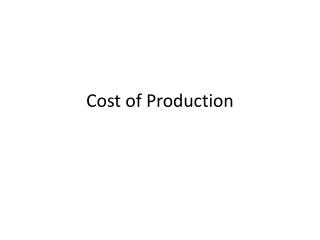DownloadDownload PresentationCost of Production

Cost of Production

Télécharger la présentationCost of Production

- - - - - - - - - - - - - - - - - - - - - - - - - - - E N D - - - - - - - - - - - - - - - - - - - - - - - - - - -
Presentation Transcript

1. Cost of Production

2. Total Revenue (TR) • Total amount a firm receives for the sale of its output • Total Cost (TC) • Total amount a firm pays for the inputs to produce its output • Profit (∏) • Total Revenue minus total cost • ∏ = TR - TC

3. Type of Cost • Accounting Cost verses Economic Cost • Explicit Cost • Actual physical payments • Implicit Cost • Opportunity costs (such as forgone interest) • Invest in building, could have invested in bonds • Economic Cost • Explicit + Implicit Costs • Accounting Cost • Only Explicit Costs

4. Economic Profit • Total Revenue minus Implicit and Explicit Costs • Accounting Profit • Total Revenue minus only Explicit costs • Therefore Economic Profit will be less than Accounting profit

5. Different Views on Profit Total Revenue

6. Production and Cost • Production Function • Relationship between quantity of inputs and quantity of output (output is good produced) • Think at Margin • Marginal Product • How much product produced due to next increment of inputs used • Diminishing Marginal Product • Marginal product declines as quantity of inputs increases • So Production Function Curve gets flatter

7. Total Cost Curve • Represents relationship between quantity of output produced and total cost to produce it • So Cost curve gets steeper • Why? Because of decreasing marginal product • Since takes more input to produce latter units of output it also costs more

8. Production Function and Costs of Production: Brownie Factory

9. Production Function and Cost Curve Output Cost \$ # of Workers Output (Quantity)

10. Types of Costs • Fixed Cost • Does not vary with quantity of output • Variable Cost • Varies with quantity of output produced • Average Fixed Cost • Fixed cost divided by quantity of output • Average Variable Cost • Variable Cost divided by quantity of output • Marginal Cost • Cost of producing one more (next) unit of output

11. Different Types of Costs of Coffee Producer \$0.30 0.50 0.70 0.90 1.10 1.30 1.50 1.70 1.90 2.10

12. Cost \$ Output (Cups of Coffee)

13. Ways of looking at cost of a unit of output • Average Total Cost (ATC) • Total cost divided by quantity • ATC = TC/Q • Cost of a typical unit • If all cost divided evenly over all output produced • Marginal Cost (MC) • Increase in total cost from one more unit output • Change in total cost divided by change in quantity • MC = ΔTC/ΔQ • Cost of one more unit

14. Shapes of Cost Curves • Rising Marginal Cost (maybe falling at first) • Due to decreasing marginal product • Become less efficient so next unit costs more • U shaped Average Total Cost • ATC = AFC + AVC • AFC always declining as output rises (1st part of U) • AVC trends up because of decreasing marginal product (2nd part of U) • Bottom of U curve • Minimizes average total cost • The point where rising AVC overtakes decline in AFC

15. Efficient Scale • Point where ATC is minimized • Relationship between MC and ATC • When MC < ATC then ATC is falling (why) • When MC > ATC then ATC is rising (why) • MC crosses ATC at its minimum point

16. Cost Curves Cost MC Why does MC cross ATC and AVC at their lowest point? Why does AVC converge to ATC? ATC AVC Why does AFC decline to zero? AFC Quantity of Output

17. Long Run vs. Short Run • Many decisions fixed in the short run but variable in the long run • Ex. Plant size, plant location, production process • Greater flexibility in the long run (can’t be worse off, only better) • Long Run Cost Curves • Differ from SR cost curves • Much flatter • Short Run Cost Curves • Lie on or above LR cost curves

18. ATC in Long Run and Short Run (U Shape for Different Reasons) Short Run ATC with small plant Cost Short Run ATC with large plant Short Run ATC with medium plant Long Run ATC Economies of Scale Diseconomies of Scale Quantity Constant Returns to Scale

19. Economies of Scale • Long Run ATC falls as quantity of output is increased • Due to increased specialization • Constant Returns to Scale • Long Run ATC stays the same as the quantity of output changes • Diseconomies of Scale • Long Run ATC increases as quantity of output is increased • Coordination problems

20. Summary of Costs